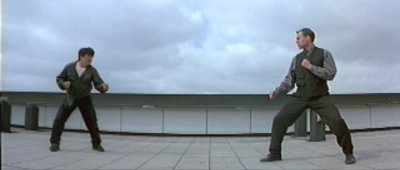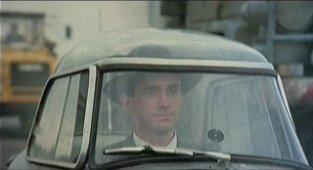Main | Jackie Chan | Jet Li | Widescreen | My Links | About | Updates | Email meBuy From:In House:## Aspect Ratio

Aspect ratio is the ratio between width vs. height. If an original image displayed on a movie screen is 235 ft. wide and 100 ft. high, it would have an aspect ratio of 2.35 to 1. Reduce the fraction of width over height: 235/100 = 2.35/1. Some other common aspect ratios include 1.33:1 and 1.78:1. These are sometimes referred to in whole number ratios as 4:3 and 16:9 respectively. 4:3 just means the display is 4 units wide for very 3 units high (or 1.33 units wide for every 1 units high). 4:3 is the ratio of a standard TV (NTSC), 16:9 is the ratio of widescreen TV's (including HDTV).

Shown below are several movies in thier original aspect ratios. For this example they all have the same height, and variable widths depending on the ratio. For more information on how these are actually seen on a Standard TV or a Widescreen TV please see thier respective pages.

#### Cinemascope, Panavision 2.35:1 aspect ratio:

When movies are filmed in this ratio the picture is a very wide, panoramic image. This is one of the widest ratios currently used. Ratios are decided upon by the producer or director so it can vary from movie to movie. Ratios can go higher than 2.35:1 although it is not very common.#### 1.85:1 aspect ratio:

This is another common aspect ratio used in movie theaters. Usually this is a matted process using an academy ratio film. The matted image may be either a hard or soft matte. See the Matte section for further details.#### 1.78:1 (16:9) aspect ratio:

This is the ratio used on current widescreen TV's. This is the midpoint between 1.33 and 2.35. That is this ratio maximizes both ratio's to get the most out of all ratios. This ratio is used in the new HDTV format.#### 1.66:1 aspect ratio:

This ratio is another common ratio for matte's#### Standard TV, 1.33:1 (4:3) aspect ratio:

Also known as Standard/Full Frame/Academy Ratio.© Ethan Killian 2002 | Last Updated: